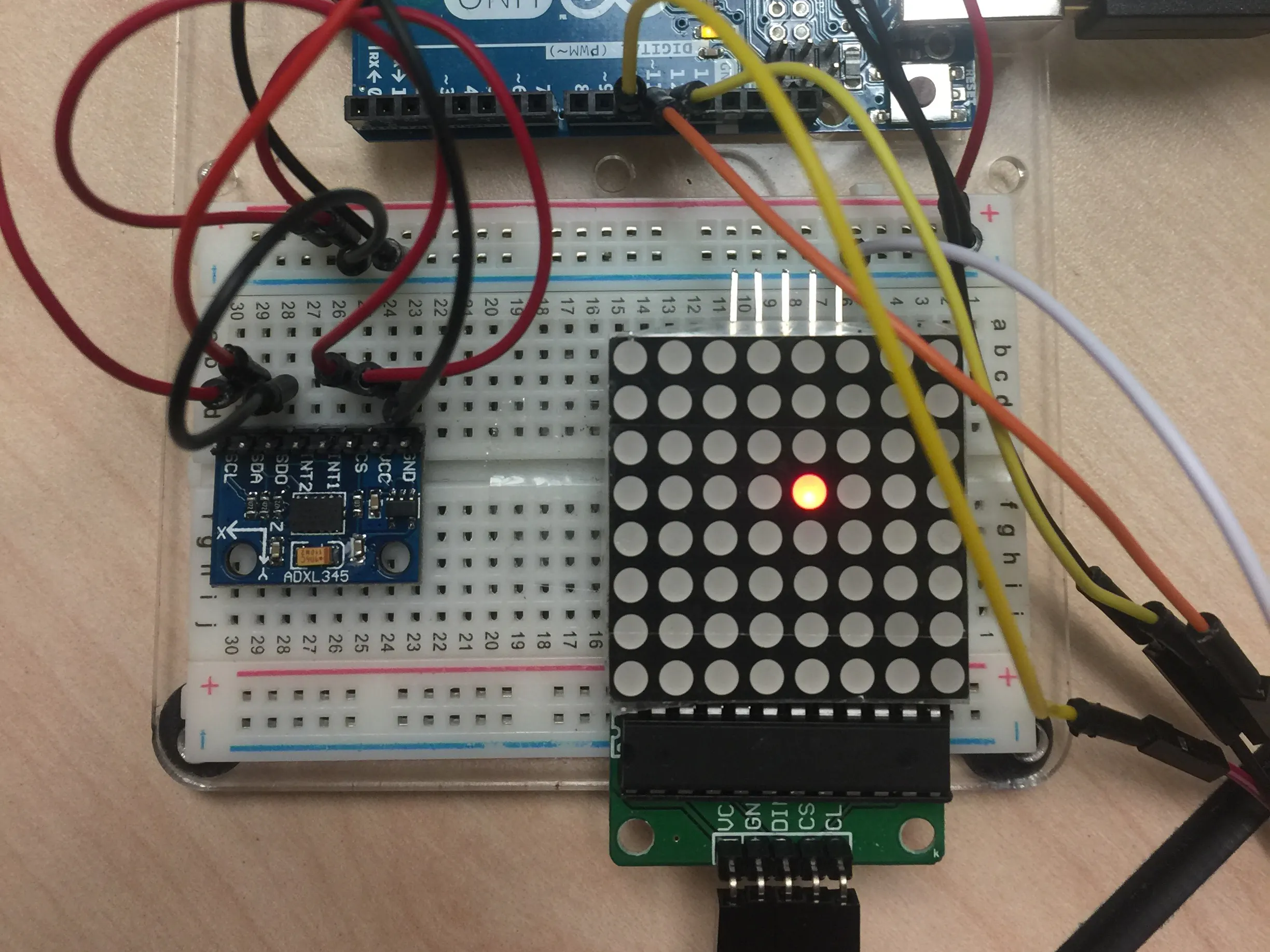Project tutorialVisual Accelerometer © GPL3+

This project connects the ADLX345 3-Axis accelerometer to display a location on an 8x8 LED matrix using the MAX7219 LED driver chip.

• 5,820 views
• 15 respects

 hands

Apps and online services

Introduction

To run this project, simply follow the wiring diagram, and then run the code in your Arduino IDE.

I made this project as a stepping stone toward larger projects that involve the ADLX345 accelerometer. This was made as a demonstration of how you can utilize both the chip, and how to display its results in the Serial monitor and physically through the LED matrix.

I decided to use the MAX7219 LED Driver to minimize the setup and the number of wires needed. It also happens to take up only 3 pins on the Arduino Uno which is a plus. As for how we orientate the matrix, unfortunately for it to follow the orientation of the accelerometer, I was not able to secure it, instead I taped it down to the board to hold it securely.

Challenges

The biggest challenge in my opinion when building this was understanding exactly what each address represents internally in the accelerometer chip. I will provide a sheet sheet that I found online that helped in setting up which addresses to READ and WRITE to.

Code

Comments are in the code - Plug it in and run it
#include <LedControl.h>
#include <Wire.h>

#define TO_READ (6)     //num of bytes we are going to read (two bytes for each axis)

byte buff[TO_READ] ;    //6 bytes buffer for saving data read from the device
char str;          //string buffer to transform data before sending it

int MATRIX_WIDTH = 8;
LedControl lc = LedControl(12, 11, 10, 1); // DIN, CLK, CS, NRDEV
unsigned long delaytime = 50;
int x_key = A1;
int y_key = A0;
int x_pos;
int y_pos;

// object that represents a single light location
// future update with gravity
class Grain
{
public:
int x = 0;
int y = 0;
int mass = 1;
};
Grain *g;

void setup()
{
// set up a grain object
g = new Grain();

ClearDisplay();

Wire.begin();        // join i2c bus (address optional for master)
Serial.begin(9600);  // start serial for output

//Turning on the ADXL345
writeTo(DEVICE, 0x2D, 0);
writeTo(DEVICE, 0x2D, 16);
writeTo(DEVICE, 0x2D, 8);
}

void loop()
{
// The first axis-acceleration-data register
int regAddress = 0x32;
int x, y, z;

// Combine the two bytes of each direction
// Least significant bit first
x = (((int)buff) << 8) | buff;
y = (((int)buff) << 8) | buff;
z = (((int)buff) << 8) | buff;

// Convert the values into values that can be represented on the matrix
x = map(x, -300, 300, 0, 8);
y = map(y, -300, 300, 0, 8);
z = map(z, -300, 300, 0, 8);

//we send the x y z values as a string to the serial port
Serial.print("X: ");
Serial.print(x);
Serial.print("   Y: ");
Serial.print(y);
Serial.print("   Z: ");
Serial.print(z);
Serial.print("\n");

ClearDisplay();
// assign the grain to this location
g->x = x;
g->y = y;
lc.setLed(0, g->x, g->y, true);

//add some delay between each update
delay(10);
}

void ClearDisplay()
{
// sets up the lcd display
int devices = lc.getDeviceCount();

{
}
}

//Writes val to address register on device
void writeTo(int device, byte address, byte val)
{
Wire.beginTransmission(device); //start transmission to device
Wire.write(val);        // send value to write
Wire.endTransmission(); //end transmission
}

//reads num bytes starting from address register on device in to buff array
void readFrom(int device, byte address, int num, byte buff[])
{
Wire.beginTransmission(device); //start transmission to device
Wire.endTransmission(); //end transmission

Wire.beginTransmission(device); //start transmission to device
Wire.requestFrom(device, num);    // request 6 bytes from device

int i = 0;
while (Wire.available())   //device may send less than requested (abnormal)
{
buff[i] = Wire.read(); // receive a byte
i++;
}
Wire.endTransmission(); //end transmission
}

Schematics

*** USE the MAX7219 Matrix (5 pin) ***Level Platform Using Accelerometer

Project tutorial by Mark Tashiro

• 23,053 views
• 36 respects

8×8 LED and Accelerometer

Project tutorial by Brink.IO

• 1,775 views
• 4 respects

Light Sequence Creator

Project tutorial by Reid Paulhus

• 10,110 views
• 22 respects

Arduino Smart Lamp

Project tutorial by Arduino “having11” Guy

• 7,314 views
• 12 respects

• 4,366 views